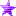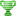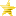# All things Variables. A Variable Tutorial (Read 19288 times)

Started by RajaaBoy, June 05, 2011, 02:30:59 pm

#### Palette Jesus##### All things Variables. A Variable Tutorial
New #1  June 05, 2011, 02:30:59 pm
••• I will absolve you of your original colors.
This tutorial is about using variables in mugen. If you don't know what a trigger is, don't read this tutorial. If you don't know what a statedef is, don't read this tutorial. If you don't know how to set up a command in the .cmd file and make a changestate to the move you want to do, then don't read this tutorial. If you don't know what the -1, -2, and -3 statedefs do, then don't read this tutorial. If you don't know what "persistent" does, don't read this tutorial. If you don't know what != means, then don't read this tutorial. If you don't know what mugen is. Then. Don't. Even. Bother. Reading. This. Tutorial.

What is a Variable?
- A variable is an entity that contains manually defined, numeric values that can be changed using the variable state controllers.
- Variable state controllers are: Varset, Varadd, Varrandom, Varrangeset.
- Read the documentation on the above state controllers.
- If they don't make any sense now, they will at the end of this tutorial.

The Use of Variables
The first thing you must know is what a variable is used for. Think of a variable as a USB storage device: You save some information to the USB device so you can use it later on, even on another computer; likewise, you save some information to your variable so that it may be used at later times, even in different statedefs (If you don't know what a statedef is, then stop reading this tutorial).

Variables contain numeric values, but that doesn't mean that's all they can represent. Later in this tutorial, I will tell you how to use variables in a more advanced way, but for now, you still want to know how to define a variable and what would be the point of defining a variable in the first place! Let's move on.

Different Types of Variables
There are two types of variables: Regular variables and float variables.

Regular variable = var(0)
Float variable = fvar(0)

There are a maximum of 60 regular variables: 0 - 59.
There are a maximum of 40 float variables: 0 - 39.

A regular variable's value cannot be a decimal of any kind, not without errors.
A float variable's value can be a decimal of any kind.

Each of those individual variables carry their own values, so changing one won't change any of the others; var(0), var(1), var(38), fvar(12) fvar(23) all contain different values. And also note that var(0) and fvar(0) are completely different and defining one won't change the other. We'll get to defining variables right now.

Defining Variables
Defining variables is the easy part! To define a variable, you just need to use one of the variable state controllers! I will tell you how to define a variable using all of the state controllers. You can test these examples out by using displaytoclipoard, I suggest you do so. Anyway, here we go:

Varset
First up is Varset. Varset sets a variable to a specific value when the triggers say so, like so:

Code:
`[State 1000]Type = VarSetTrigger1 = Time = 10V  = 0Value = 1`

The above code will set variable 0 to 1 when the time of the current state is equal to 10.

Here is a more practical way to use the varset state controller, and I suggest getting used to this way, it saves space and is more logical:

Code:
`[State 1000]Type = VarSetTrigger1 = Time = 10Var(0) = 1`

Now, var(0) is equal to 1. You've set your variable. Of course, you will need to use your own triggers for how you want to set the variables, but you should get the idea from this example.

Now, lets go on to Varadd. Varadd, instead of completely shifting the value of a variable to the value specified, adds the amount specified to the current value of the variable. Here's an example:

Code:
`[State 1000]Type = VarAddTrigger1 = Time = 15Var(0) = 5`

Now, above in the varset example, the varset state controller set var(0) to 1 when the state time was 10; now the state time is 15 and a varadd is being activated. The above code, instead of changing var(0) from 1 to 5, adds 5 to 1, and now var(0) is equal to 6! I guess this is the time to mention that all variables' values default to 0, so if you never defined a variable before you used a varadd, it'll just be adding the value specified to 0.

Varrandom
Next up is Varrandom. Varrandom is exactly what it sounds like, it takes a variable and sets it to a random value within the specified range:

Code:
`[State 1000]Type = VarRandomTrigger1 = Time = 20V = 0Range = 10, 20`

Even though, var(0) was 6 before, when the above state controller activates, it will be set to any value from 10 to 20, including 10 and 20. It sets, it doesn't add. This is self explanatory and the documentation should have already cleared this up for you.

Varrangeset
Finally, there is Varrangeset. Varrangeset sets a group of variables to a specific value. Here's your example:

Code:
`[State 1000]Type = VarRangeSetTrigger1 = Time= 25first = 0last = 5value = 100`

That will set all variables from 0 to 5, including 0 and 5, to 100.

Now you know how to use the various variable sate controllers, now you need a reason to use them. Next step is me showing you how to put good use to your variables.

Putting Variables to Good Use
The first thing you must know about a variable is that to put it to good use is to not have to use it all! Variables are currently limited in mugen, no need to waste them if you don't have to.

Using variables as triggers is the point of this section, the triggers, of course, will be based off of the values of the variable. Here's a simple example that will settle your confusion of how to use variables once and for all:

Code:
`[Statedef 1000][State 1000]Type = VarSetTrigger1 = Time = 0Var(0) = 1[State 1000, Variable Based Animation]Type = ChangeAnimTrigger1 = var(0) = 1Value = 1000Persistent = 0[State 1000, Variable Based Animation]Type = ChangeAnimTrigger1 = var(0) != 1Value= 1005Persistent = 0`

The above code is simple if you've been following this tutorial correctly. At the start of statedef 1000 there is a varset state controller that sets var(0) to 1. The two changeanim state controllers trigger based on the value of var(0), in this case, the animation that will be played is 1000 since var(0) is equal to 1. If you want to toy around with the above code, kindly place it in a test statedef, change the values of the changeanim state controllers to animations your character already has, and then change the varset state controller's value from 1 to 0, and from 0 back to 1, and you will see which animation gets triggered when.

That's how you efficiently use a variable.

!= means "Not equal to," in case you read this tutorial anyway after I told you not to read it if you didn't know what that meant

How to Stop your Variables from Resetting between Rounds
In your character's constants, you should see these two lines:

IntPersistIndex = 60
FloatPersistIndex = 40

Any variable equal to and above those values will not be reset between rounds (integer and float respectively). So if you don't want any of your variables reset, you can just change those values to 0. You know what you want, there's nothing else to say here.

One More Example
One of the common ways coders use variables is for alternate modes activated during a match.

So, if you want your character to turn evil during a match, you would set up a move that takes you to the statedef where the transforming pose is done, and in that state, you will set a variable to a value that represents that your character has turned evil.

So, in that statedef you will have:

Code:
`[State 4000, Evil Variable]Type = VarSetTrigger1 = Time = 0Var(50) = 1`

That sets var(50) to 1. Now you can use var(50) to trigger things that should and shouldn't be activated while in evil mode! Here's a slight modification of a previous example so you can get the idea:

Code:
`[Statedef 1000] ; Fireball[State 1000, Normal Fireball Animation]Type = ChangeAnimTrigger1 = var(50) != 1Value = 1000Persistent = 0[State 1000, Evil Fireball Animation]Type = ChangeAnimTrigger1 = var(50) = 1Value = 1005Persistent = 0`

Needless to say, if you already turned evil before changing to the above statedef, then your character's var(50) would equal 1, and because of that, the evil fireball animation would play instead of the normal fireball animation that would play if you didn't turn evil and change your character's var(50) to 1.

Setting Variables without a Varset
This is pretty tricky, but gets the job done in many cases once you get used to it.

Now, say you want to set a variable when certain state controllers activate, but you don't feel like making a brand new varset state controller for each state controller you need to set your variable for -- well, there's good news for you, you can set the variable as so without loading your code with a bunch of varset sate controllers:

Code:
`[State 1000]Type = ChangeAnimTrigger1 = Time = 0*****Value = Var(0) := 1000*****`

:= means "set this variable to." Note that the value chosen to set the variable to is not boolean and will actually be read as "1000" by mugen. "Boolean," for purposes of mugen, means: mugen either reads the value as 0 or 1; no, or yes.

Anyway, the above code activates at time = 0 and then it sets var(0) to 1000 while at the same time changing to animation 1000. As explained above, that isn't a boolean expression, so mugen actually reads it as 1000 which allows that to work.

One reason you might want to set the variable that way is so you can keep track of what animation your character changes to each time a changeanim is activated, use your imagination to think of the things that are possible by means of setting a variable that way.

You can even use it as a trigger:

Code:
`[State 1000]Type = ChangeAnimTrigger1 = Time = 0Trigger1 = Var(0) := 1000Value = 1000`
Note, that if you use it as a trigger, if the variable is being set to 0, the whole set of triggers won't activate because a trigger of 0 means "don't activate" to Mugen, and I already explained the "boolean thing."

Some Variable Tips
1. Don't use a variable if you don't have to. Using many variables could get confusing to you and it's just not even worth wasting them. If you need to use variables, make sure to jot down their usage for yourself and others who might want to edit your character.

2. Helpers have their own variables! Don't be afraid to use helpers for variables. If you know how to use redirection triggers, then using helpers to harness variables is not only convenient, you essentially have tons more variables at your disposal. Remember, though, just because you can, doesn't mean you should, and reading helpers' variables is slow by 1 tick in some cases, so it might not work as best as you may want.

3. Remember that var and fvar are different, but they are interchangeable between every method I described above!

It's Only as Good as Your Will to Learn

This tutorial is only as good as your ability to experiment, learn and understand. If you aren't willing to take the time it takes to become comfortable with anything pertaining to coding mugen characters, then you're not ever going to get comfortable with coding mugen characters.

Cheers, Rajaa.

Last Edit: December 06, 2016, 03:46:19 am by Just No Point